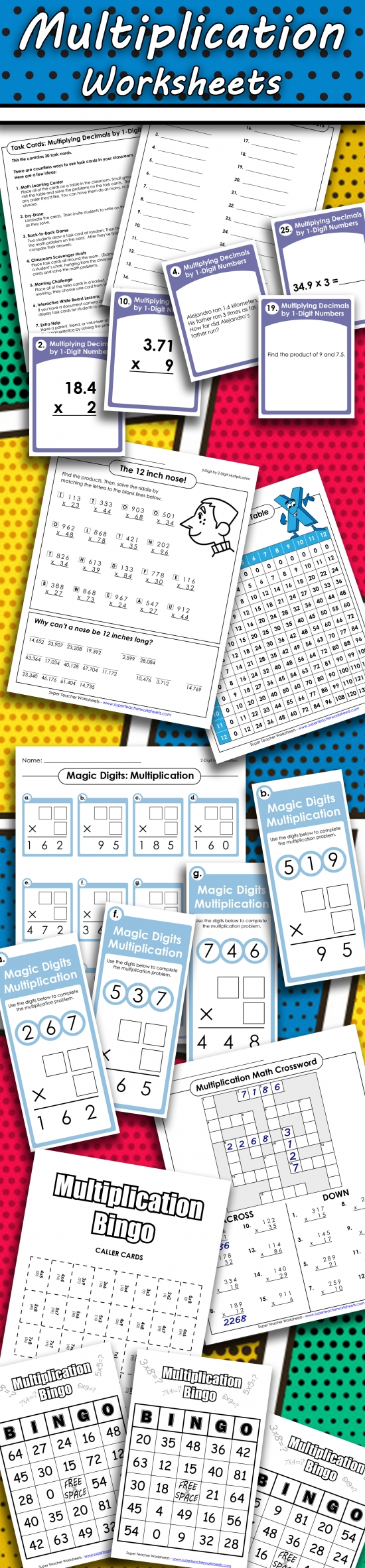# Multiplication ResourcesSuper Teacher Worksheets has a plethora of multiplication resources for basic and advanced multiplication skills. Whether you're looking to cover multiplication facts, fact families, multiplication tables, properties of multiplication, multi-digit multiplication, multiplying money, multiplying decimals, or multiplying fractions, we have the resources you need! You'll find worksheets, drills, games, task cards, classroom activities, math riddles, crossword puzzles, math mystery pictures, and much more! Take a look at our multiplication worksheets collection today!